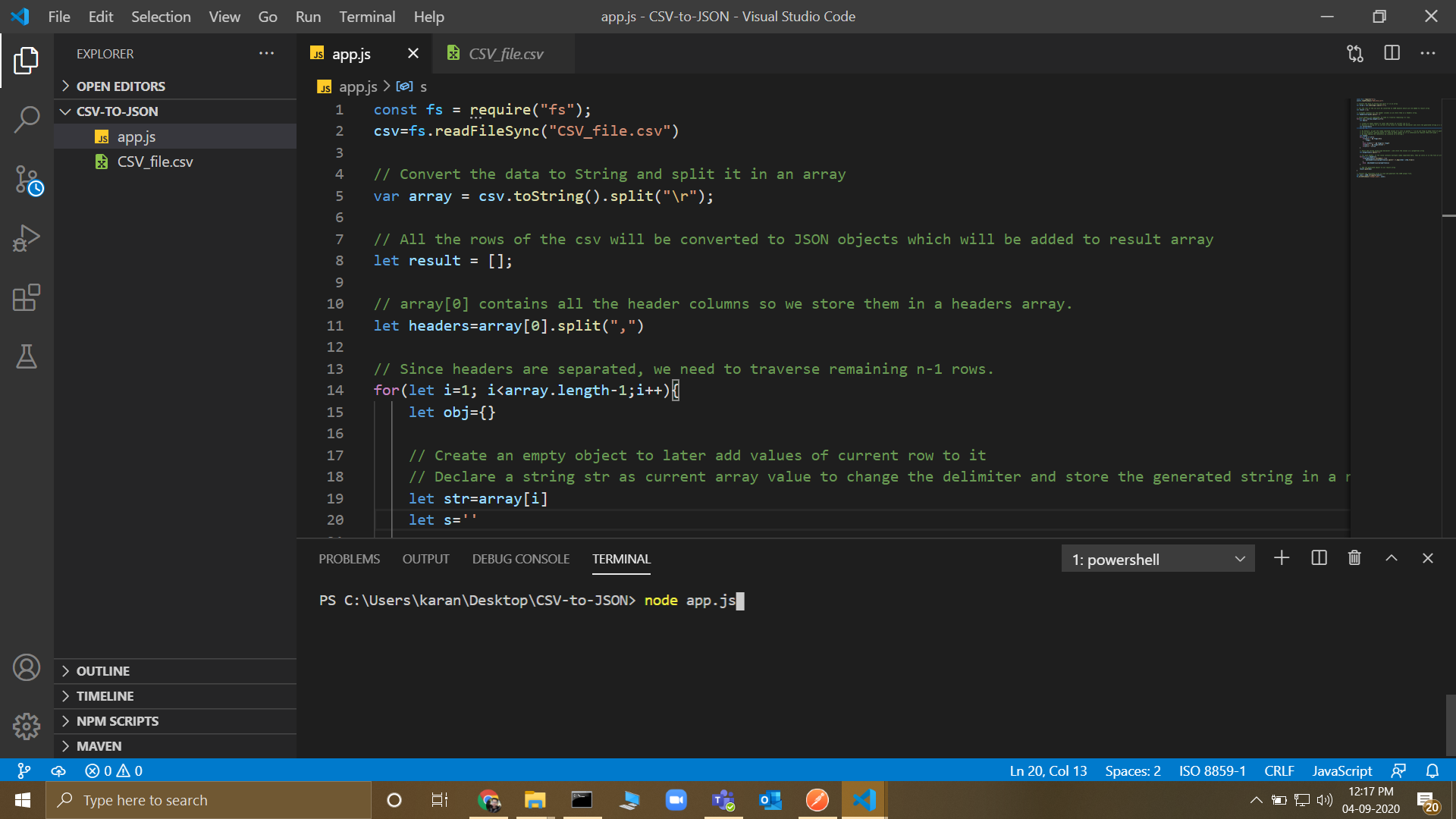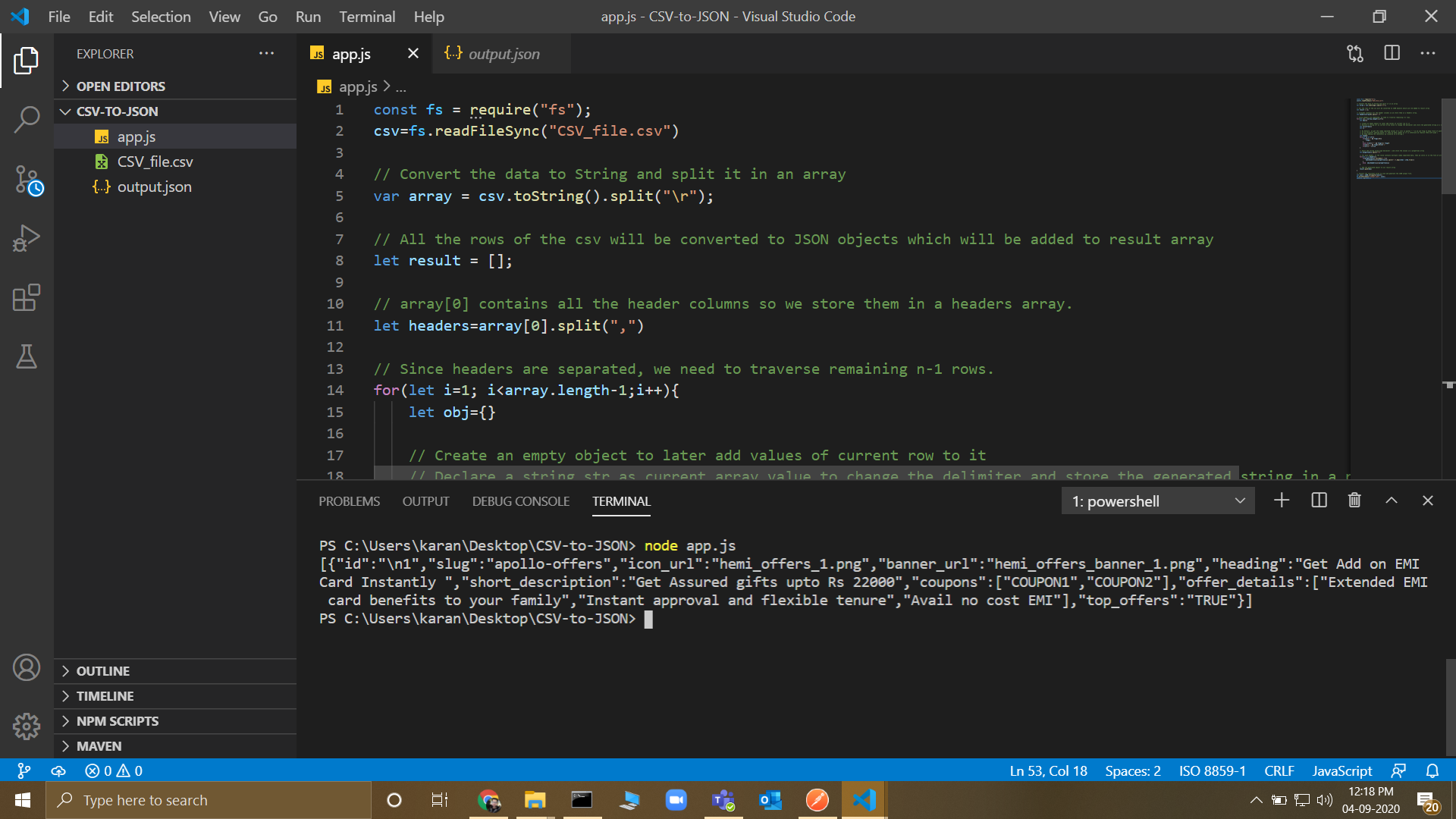Related Articles

# How to Convert CSV to JSON file having Comma Separated values in Node.js ?

• Last Updated : 19 May, 2021

A CSV is a comma-separated values file with .csv extension, which allows data to be saved in a tabular format. Here is a article to convert the data of a csv file to a JavaScript Object Notation (JSON) without using any third party npm package. The main difference from normal conversion is that the values of any row can be Comma Separated and as we know, different columns are also comma separated.

In this approach, we will input the contents of the CSV file in an array and split the content of the array based on a delimiter. All the rows of the CSV will be converted to JSON objects which will be added to the resultant array which will then be converted to JSON and a corresponding JSON output file will be generated.

Approach:
Follow the steps below to achieve the solution:

1. Read the csv file using default fs npm package.
2. Convert the data to String and split it in an array.
4. For all the remaining n-1 rows do the following:
• Create an empty object to add values of current row to it.
• Declare a string str as current array value to change the delimiter and store the generated string in a new string s.
• If we encounter opening quote (“) then we keep commas as it is otherwise we replace them with pipe “|”
• Keep adding the characters we traverse to a String s.
• Split the string using pipe delimiter | and store the values in a properties array.
• For each header, if the value contains multiple comma separated data, then we store it in the form of array otherwise directly the value is stored.
• Add the generated object to our result array.
5. Convert the resultant array to json and generate the JSON output file.

Filename: app.js

## javascript

 `// Reading the file using default``// fs npm package``const fs = require(``"fs"``);``csv = fs.readFileSync(``"CSV_file.csv"``)` `// Convert the data to String and``// split it in an array``var` `array = csv.toString().split(``"\r"``);` `// All the rows of the CSV will be``// converted to JSON objects which``// will be added to result in an array``let result = [];` `// The array contains all the``// header columns so we store them``// in headers array``let headers = array.split(``", "``)` `// Since headers are separated, we``// need to traverse remaining n-1 rows.``for` `(let i = 1; i < array.length - 1; i++) {``  ``let obj = {}` `  ``// Create an empty object to later add``  ``// values of the current row to it``  ``// Declare string str as current array``  ``// value to change the delimiter and``  ``// store the generated string in a new``  ``// string s``  ``let str = array[i]``  ``let s = ``''` `  ``// By Default, we get the comma separated``  ``// values of a cell in quotes " " so we``  ``// use flag to keep track of quotes and``  ``// split the string accordingly``  ``// If we encounter opening quote (")``  ``// then we keep commas as it is otherwise``  ``// we replace them with pipe |``  ``// We keep adding the characters we``  ``// traverse to a String s``  ``let flag = 0``  ``for` `(let ch of str) {``    ``if` `(ch === ``'"'` `&& flag === 0) {``      ``flag = 1``    ``}``    ``else` `if` `(ch === ``'"'` `&& flag == 1) flag = 0``    ``if` `(ch === ``', '` `&& flag === 0) ch = ``'|'``    ``if` `(ch !== ``'"'``) s += ch``  ``}` `  ``// Split the string using pipe delimiter |``  ``// and store the values in a properties array``  ``let properties = s.split(``"|"``)` `  ``// For each header, if the value contains``  ``// multiple comma separated data, then we``  ``// store it in the form of array otherwise``  ``// directly the value is stored``  ``for` `(let j ``in` `headers) {``    ``if` `(properties[j].includes(``", "``)) {``      ``obj[headers[j]] = properties[j]``        ``.split(``", "``).map(item => item.trim())``    ``}``    ``else` `obj[headers[j]] = properties[j]``  ``}` `  ``// Add the generated object to our``  ``// result array``  ``result.push(obj)``}` `// Convert the resultant array to json and``// generate the JSON output file.``let json = JSON.stringify(result);``fs.writeFileSync(``'output.json'``, json);`

Input:Run the command “node app.js” on the terminal to run the program.

Output:The output.json file gets created and the content of the file has been loggeed on the terminal.

My Personal Notes arrow_drop_up